sbiopredictionci

Compute confidence intervals for model predictions (requires Statistics and Machine Learning Toolbox)

Description

example

ci = sbiopredictionci(fitResults) computes 95% confidence intervals for the model simulation results from fitResults, an NLINResults object or OptimResults object returned by sbiofit. ci is a PredictionConfidenceInterval object that contains the computed confidence interval data.

ci = sbiopredictionci(fitResults,Name,Value) uses additional options specified by one or more Name,Value pair arguments.

Examples

collapse all

Load the sample data to fit. The data is stored as a table with variables ID , Time , CentralConc , and PeripheralConc. This synthetic data represents the time course of plasma concentrations measured at eight different time points for both central and peripheral compartments after an infusion dose for three individuals.

gData = groupedData(data);
gData.Properties.VariableUnits = {'','hour','milligram/liter','milligram/liter'};
sbiotrellis(gData,'ID','Time',{'CentralConc','PeripheralConc'},'Marker','+',...
'LineStyle','none');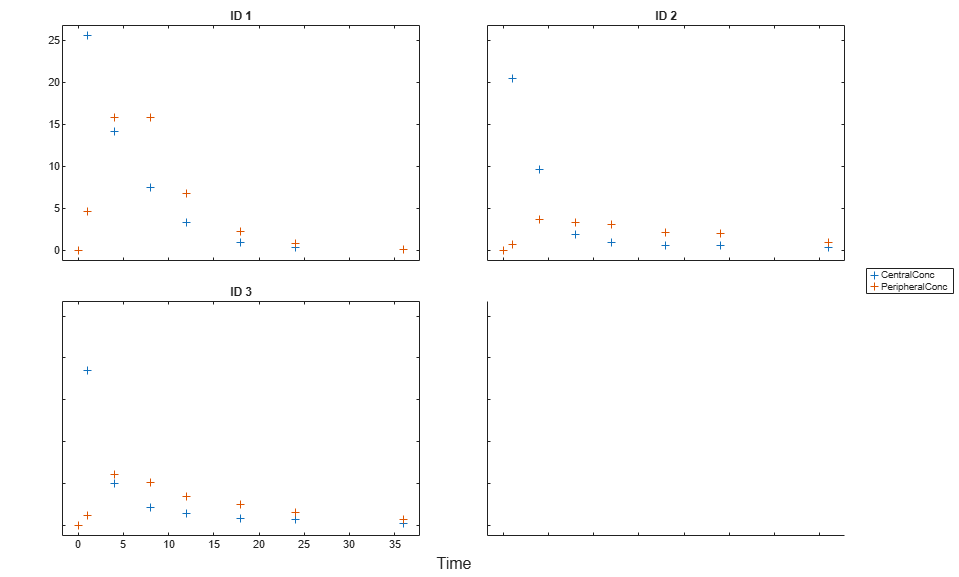Create Model

Create a two-compartment model.

pkmd                 = PKModelDesign;
pkc1.DosingType      = 'Infusion';
pkc1.EliminationType = 'linear-clearance';
pkc1.HasResponseVariable = true;
model                = construct(pkmd);
configset            = getconfigset(model);
configset.CompileOptions.UnitConversion = true;

Define Dosing

Define the infusion dose.

dose             = sbiodose('dose','TargetName','Drug_Central');
dose.StartTime   = 0;
dose.Amount      = 100;
dose.Rate        = 50;
dose.AmountUnits = 'milligram';
dose.TimeUnits   = 'hour';
dose.RateUnits   = 'milligram/hour';

Define Parameters

Define the parameters to estimate. Set the parameter bounds for each parameter. In addition to these explicit bounds, the parameter transformations (such as log, logit, or probit) impose implicit bounds.

responseMap = {'Drug_Central = CentralConc','Drug_Peripheral = PeripheralConc'};
paramsToEstimate   = {'log(Central)','log(Peripheral)','Q12','Cl_Central'};
estimatedParam     = estimatedInfo(paramsToEstimate,...
'InitialValue',[1 1 1 1],...
'Bounds',[0.1 3;0.1 10;0 10;0.1 2]);

Fit Model

Perform an unpooled fit, that is, one set of estimated parameters for each patient.

unpooledFit = sbiofit(model,gData,responseMap,estimatedParam,dose,'Pooled',false);

Perform a pooled fit, that is, one set of estimated parameters for all patients.

pooledFit = sbiofit(model,gData,responseMap,estimatedParam,dose,'Pooled',true);

Compute Confidence Intervals for Estimated Parameters

Compute 95% confidence intervals for each estimated parameter in the unpooled fit.

ciParamUnpooled = sbioparameterci(unpooledFit);

Display Results

Display the confidence intervals in a table format. For details about the meaning of each estimation status, see Parameter Confidence Interval Estimation Status.

ci2table(ciParamUnpooled)
ans =

12x7 table

Group         Name         Estimate    ConfidenceInterval      Type      Alpha      Status
_____    ______________    ________    __________________    ________    _____    ___________

1      {'Central'   }      1.422      1.1533     1.6906    Gaussian    0.05     estimable
1      {'Peripheral'}     1.5629     0.83143     2.3551    Gaussian    0.05     constrained
1      {'Q12'       }    0.47159     0.20093    0.80247    Gaussian    0.05     constrained
1      {'Cl_Central'}    0.52898     0.44842    0.60955    Gaussian    0.05     estimable
2      {'Central'   }     1.8322      1.7893     1.8751    Gaussian    0.05     success
2      {'Peripheral'}     5.3368      3.9133     6.7602    Gaussian    0.05     success
2      {'Q12'       }    0.27641      0.2093    0.34351    Gaussian    0.05     success
2      {'Cl_Central'}    0.86034     0.80313    0.91755    Gaussian    0.05     success
3      {'Central'   }     1.6657      1.5818     1.7497    Gaussian    0.05     success
3      {'Peripheral'}     5.5632      4.7557     6.3708    Gaussian    0.05     success
3      {'Q12'       }    0.78361     0.65581    0.91142    Gaussian    0.05     success
3      {'Cl_Central'}     1.0233     0.96375     1.0828    Gaussian    0.05     success

Plot the confidence intervals. If the estimation status of a confidence interval is success, it is plotted in blue (the first default color). Otherwise, it is plotted in red (the second default color), which indicates that further investigation into the fitted parameters may be required. If the confidence interval is not estimable, then the function plots a red line with a centered cross. If there are any transformed parameters with estimated values 0 (for the log transform) and 1 or 0 (for the probit or logit transform), then no confidence intervals are plotted for those parameter estimates. To see the color order, type get(groot,'defaultAxesColorOrder').

Groups are displayed from left to right in the same order that they appear in the GroupNames property of the object, which is used to label the x-axis. The y-labels are the transformed parameter names.

plot(ciParamUnpooled)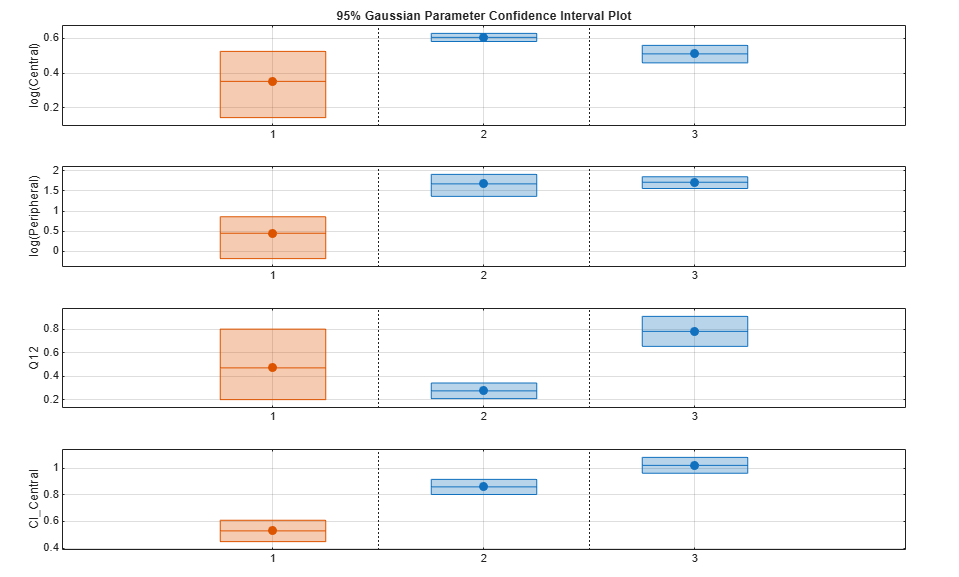Compute the confidence intervals for the pooled fit.

ciParamPooled = sbioparameterci(pooledFit);

Display the confidence intervals.

ci2table(ciParamPooled)
ans =

4x7 table

Group          Name         Estimate    ConfidenceInterval      Type      Alpha      Status
______    ______________    ________    __________________    ________    _____    ___________

pooled    {'Central'   }     1.6626      1.3287     1.9965    Gaussian    0.05     estimable
pooled    {'Peripheral'}      2.687     0.89848     4.8323    Gaussian    0.05     constrained
pooled    {'Q12'       }    0.44956     0.11445    0.85152    Gaussian    0.05     constrained
pooled    {'Cl_Central'}    0.78493     0.59222    0.97764    Gaussian    0.05     estimable

Plot the confidence intervals. The group name is labeled as "pooled" to indicate such fit.

plot(ciParamPooled)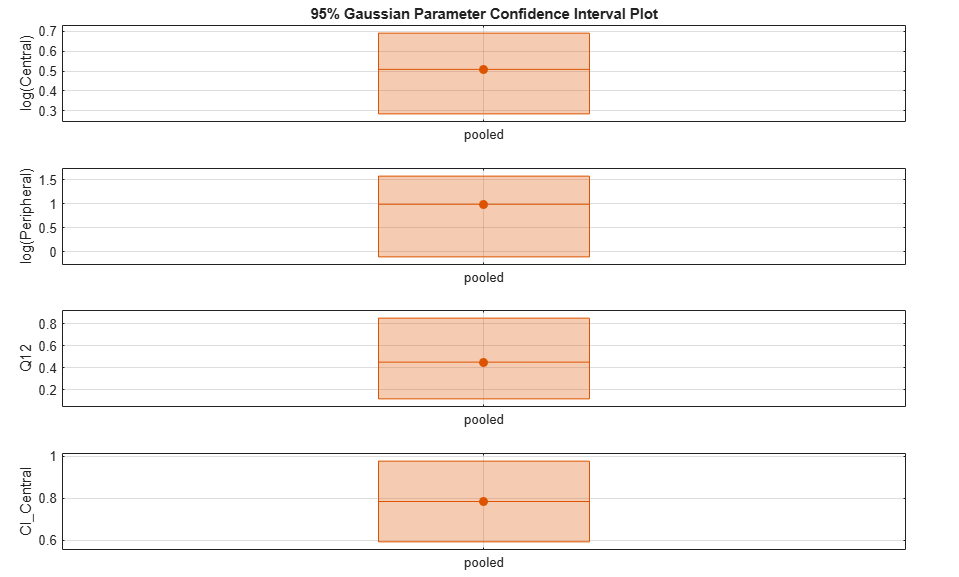Plot all the confidence interval results together. By default, the confidence interval for each parameter estimate is plotted on a separate axes. Vertical lines group confidence intervals of parameter estimates that were computed in a common fit.

ciAll = [ciParamUnpooled;ciParamPooled];
plot(ciAll)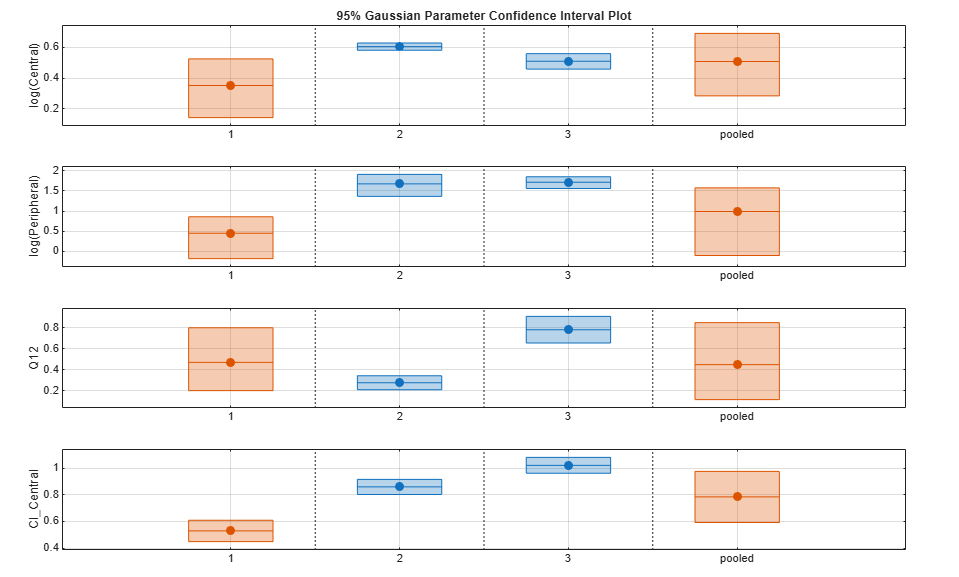You can also plot all confidence intervals in one axes grouped by parameter estimates using the 'Grouped' layout.

plot(ciAll,'Layout','Grouped')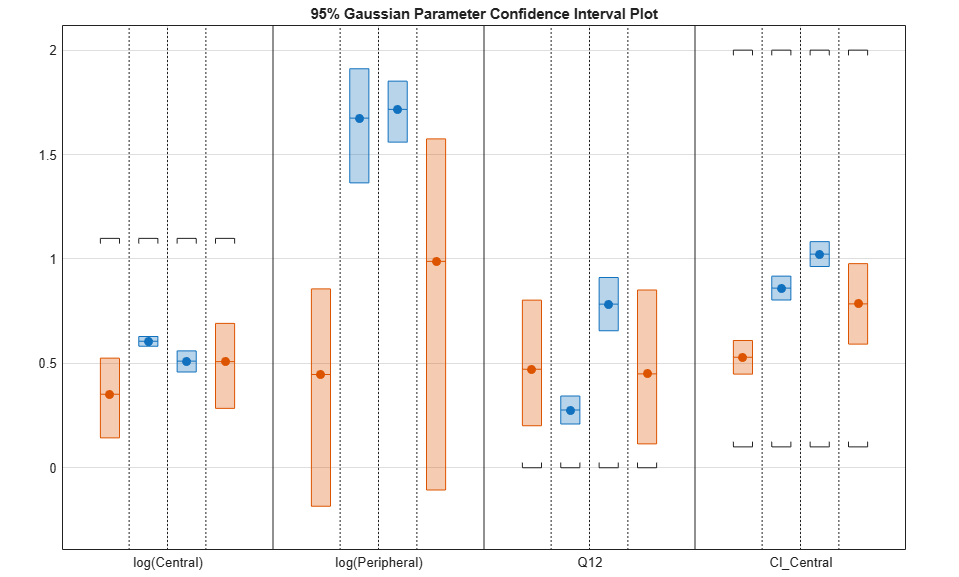In this layout, you can point to the center marker of each confidence interval to see the group name. Each estimated parameter is separated by a vertical black line. Vertical dotted lines group confidence intervals of parameter estimates that were computed in a common fit. Parameter bounds defined in the original fit are marked by square brackets. Note the different scales on the y-axis due to parameter transformations. For instance, the y-axis of Q12 is in the linear scale, but that of Central is in the log scale due to its log transform.

Compute Confidence Intervals for Model Predictions

Calculate 95% confidence intervals for the model predictions, that is, simulation results using the estimated parameters.

% For the pooled fit
ciPredPooled = sbiopredictionci(pooledFit);
% For the unpooled fit
ciPredUnpooled = sbiopredictionci(unpooledFit);

Plot Confidence Intervals for Model Predictions

The confidence interval for each group is plotted in a separate column, and each response is plotted in a separate row. Confidence intervals limited by the bounds are plotted in red. Confidence intervals not limited by the bounds are plotted in blue.

plot(ciPredPooled)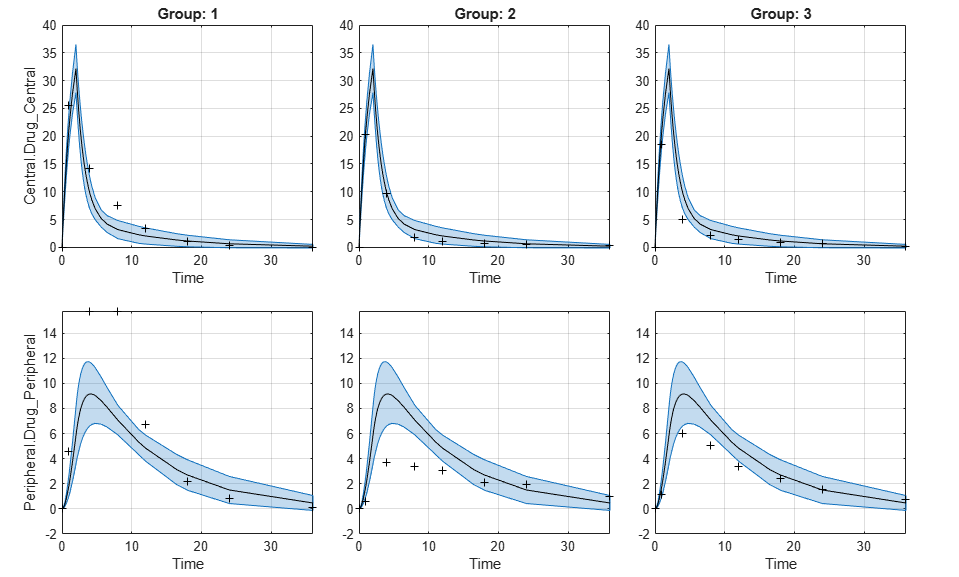plot(ciPredUnpooled)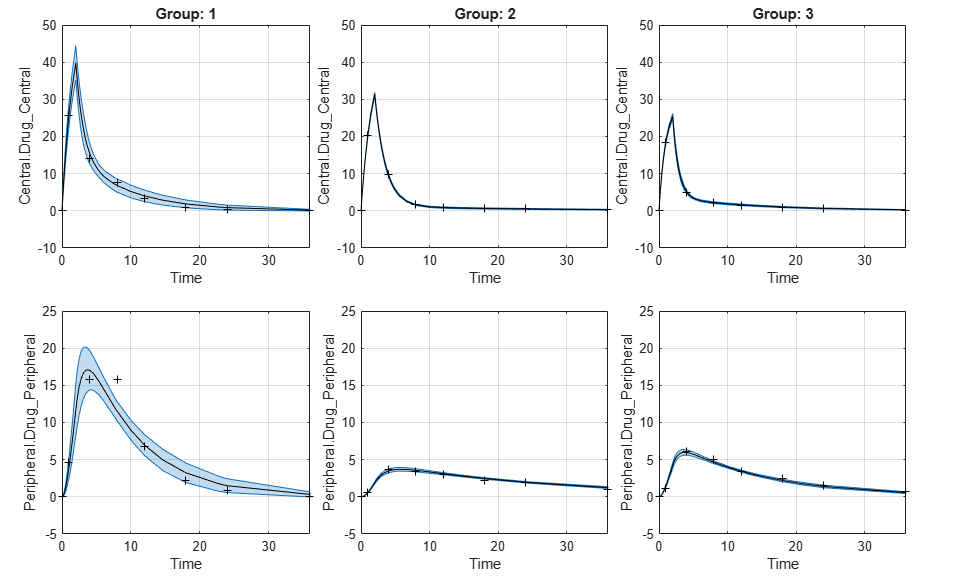Input Arguments

collapse all

Parameter estimation results from sbiofit, specified as an NLINResults object, OptimResults object, or a vector of objects for unpooled fits that were returned from the same sbiofit call.

Name-Value Arguments

Specify optional comma-separated pairs of Name,Value arguments. Name is the argument name and Value is the corresponding value. Name must appear inside quotes. You can specify several name and value pair arguments in any order as Name1,Value1,...,NameN,ValueN.

Example: 'Alpha',0.01,'Type','bootstrap' specifies to compute a 99% confidence interval using the bootstrap method.

Confidence level, (1-Alpha) * 100%, specified as the comma-separated pair consisting of 'Alpha' and a positive scalar between 0 and 1. The default value is 0.05, meaning a 95% confidence interval is computed.

Example: 'Alpha',0.01

Confidence interval type, specified as the comma-separated pair consisting of 'Type' and a character vector. The valid choices are:

Example: 'Type','bootstrap'

Number of samples for bootstrapping, specified as the comma-separated pair consisting of 'NumSamples' and a positive integer. This number defines the number of fits that are performed during the confidence interval computation to generate bootstrap samples. The smaller the number is, the faster the computation of the confidence intervals becomes, at the cost of decreased accuracy.

Example: 'NumSamples',500

Level of display returned to the command line, specified as the comma-separated pair consisting of 'Display' and a character vector. 'off' (default) or 'none' displays no output. 'final' displays a message when the computation finishes.

Example: 'Display','final'

Logical flag to compute confidence intervals in parallel, specified as the comma-separated pair consisting of 'UseParallel' and true or false. By default, the parallel options in the original fit are used. If this argument is set to true and Parallel Computing Toolbox™ is available, the parallel options in the original fit are ignored, and confidence intervals are computed in parallel.

For the Gaussian confidence intervals:

• If the input fitResults is a vector of results objects, then the computation of confidence intervals for each object is performed in parallel. The Gaussian confidence intervals are quick to compute. So, it might be more beneficial to parallelize the original fit (sbiofit) and not set UseParallel to true for sbiopredictionci.

For the Bootstrap confidence intervals:

• The function forwards the UseParallel flag to bootci. There is no parallelization over the input vector of results objects.

Note

If you have a global stream for random number generation with a number of substreams to compute in parallel in a reproducible fashion, sbiopredictionci first checks to see if the number of workers is same as the number of substreams. If so, the function sets UseSubstreams to true in the statset option and passes to bootci (Statistics and Machine Learning Toolbox). Otherwise, the substreams are ignored by default.

Example: 'UseParallel',true

Output Arguments

collapse all

Confidence interval results, returned as a PredictionConfidenceInterval object. For an unpooled fit, ci can be a vector of PredictionConfidenceInterval objects.

collapse all

Gaussian Confidence Interval Calculation for Model Predictions

The model is linearized around the parameter estimates Pest that are obtained from the fit results returned by sbiofit. The CovarianceMatrix is transformed using the linearized model. In addition, implicit parameter bounds (log, probit, or logit parameter transforms specified in the original fit) and explicit parameter bounds (if specified in the original fit) are also mapped through the linearized model.

To linearize the model, sbiopredictionci first checks to see if the sensitivity analysis feature is turned on in the original fit. If the feature is on, the function uses the Jacobian computed via the complex step differentiation. If the feature is off, the Jacobian is computed using finite differencing. Finite differencing can be inaccurate, and consider turning on the sensitivity analysis feature when you run sbiofit.

The function uses the transformed CovarianceMatrix and computes the Gaussian confidence intervals for each estimated model response at every time step.

In cases where the confidence interval is constrained by the parameter bounds defined in the original fit, the confidence interval bounds are adjusted according to the approach described by Wu, H. and Neale, M. .

Setting Estimation Status

• For each model response, the function first decides whether the confidence interval is unbounded. If so, the estimation status of the corresponding model response is set to not estimable.

• Otherwise, if the confidence interval for a response is constrained by a parameter bound defined in the original fit, the function sets its status to constrained. Parameter transformations (such as log, probit, or logit) impose implicit bounds on the estimated parameters, for example, positivity constraints. Such bounds can lead to the overestimation of confidence, that is, the confidence interval can be smaller than expected.

• If no confidence interval has the estimation status not estimable or constrained, then the function sets the estimation statuses of all model responses to success. Otherwise, the estimation statuses of remaining model responses are set to estimable.

Bootstrap Confidence Interval Calculation

The bootci (Statistics and Machine Learning Toolbox) function from Statistics and Machine Learning Toolbox™ is used to compute the bootstrap confidence intervals. The first input nboot is the number of samples (NumSamples), and the second input bootfun is a function that performs these actions.

• Resample the data (independently within each group, if multiple groups are available).

• Run a parameter fit with the resampled data.

• Simulate the model using the estimated parameters to get model responses.

• Return model responses.

Setting Estimation Status

The estimation status is always set to estimable since the function cannot determine if the confidence intervals are constrained by the bounds on the parameter estimates.

 Wu, H., and M.C. Neale. "Adjusted Confidence Intervals for a Bounded Parameter." Behavior Genetics. 42 (6), 2012, pp. 886-898.

SimBiology DocumentationGet trial now